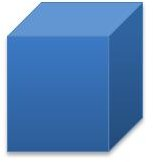# Finding the Volume of a Rectangular Prism: Lesson Plan for 6th Grade

Page content

Introduction: Find volumes of right rectangular prisms by filling prisms with unit cubes with fractional edge lengths. Students explore the connection between filling a prims with unit cubes and the volume formula.

Lesson Objective: The lesson is aligned to the Common Core State Standards for Mathematics – 6.G.2 Geometry – Find the volume of a right rectangular prism with fractional edge lengths by packing it with unit cubes of the appropriate unit fraction edge lengths, and show that the volume is the same as would be found by multiplying the edge lengths of the prism.

Materials Required: Unit Cubes, Calculator

## Lesson Procedure: Finding the Volume of a Right Rectangular Prism

Look at the right rectangular prism on the right. The measurement of the length is 2¼ inches. The measurement of the width is 1/2 inch. The measurement of the height is 1 inch.

Change the dimensions of the right rectangular prism to improper-like fractions. The dimensions are 9/4, 2/4, and 4/4 inches.

To find the volume of this right rectangular prism, fill it with ¼ inch cubes. It takes 64 – ¼ inch cubes to fill one unit cube.

The right rectangular prism can be filled with ¼ inch cubes. It takes 9 x 2 x 4 cubes to fill this right rectangular prism. It takes 72 – ¼ inch cubes to fill this right rectangular prism.

The volume of the right rectangular prism is 9/4 x 2/4 x 4/4 = 72/64 or 1 8/64 or 1 1/8 cubic inches.

## Individual or Group Work:Look at the right rectangular prisms below. Fill the prisms with unit cubes of the appropriate unit fraction edge lengths.

1. The measurement of the length is 2 inches. The measurement of the width is 1 inch. The measurement of the height is 3¾ inches. How many cubes with side lengths of ¼ would be needed to fill the prism? What is the volume of the prism? Show how you found your answers.2. The measurement of the length is 1½ inches. The measurement of the width is ½ inch. The measurement of the height is 4 inches. How many cubes with side lengths of 1/2 inch would be needed to fill the prism? What is the volume of the prism? Show how you found your answers.3. The measurement of the length is 4 1/2 inches. The measurement of the width is 1 ½ inch. The measurement of the height is 2 inches. How many cubes with side lengths of 1/2 inch would be needed to fill the prism? What is the volume of the prism? Show how you found your answers.4. The measurement of the length is 3/4 inches. The measurement of the width is ½ inch. The measurement of the height is 2 inches. How many cubes with side lengths of 1/4 inch would be needed to fill the prism? What is the volume of the prism? Show how you found your answers.5. The measurement of the length is 2½ inches. The measurement of the width is 3 ½ inch. The measurement of the height is 4 inches. How many cubes with side lengths of 1/2 inch would be needed to fill the prism? What is the volume of the prism? Show how you found your answers.

1. It takes 8 x 4 x 15 cubes, or 480 cubes with side lengths of ¼ inch. The volume of the right rectangular prism is 480/64 = 7 ½ cubic inches or 2 x 1 x 3 ¾ = 7 ½ cubic inches.

2. It takes 3 x 1 x 8 cubes, or 24 cubes with side lengths of ½ inch. The volume of the right rectangular prism is 24/8 = 3 cubic inches or 1 ½ x ½ x 4 = 3 cubic inches.

3. It takes 9 x 3 x 4 cubes, or 108 cubes with side lengths of ½ inch. The volume of the right rectangular prism is 108/8 = 13 ½ cubic inches or 4 ½ x 1 1/2 x 2 = 13 1/2 cubic inches.

4. It takes 3 x 2 x 8 cubes, or 48 cubes with side lengths of ¼ inch. The volume of the right rectangular prism is 48/64 = ¾ cubic inches or ¾ x ½ x 2 = ¾ cubic inches.

5. It takes 5 x 7 x 8 cubes, or 280 cubes with side lengths of ½ inch. The volume of the right rectnagular prism is 280/8 = 35 cubic inches or 2 ½ x 3 ½ x 4 = 35 cubic inches.